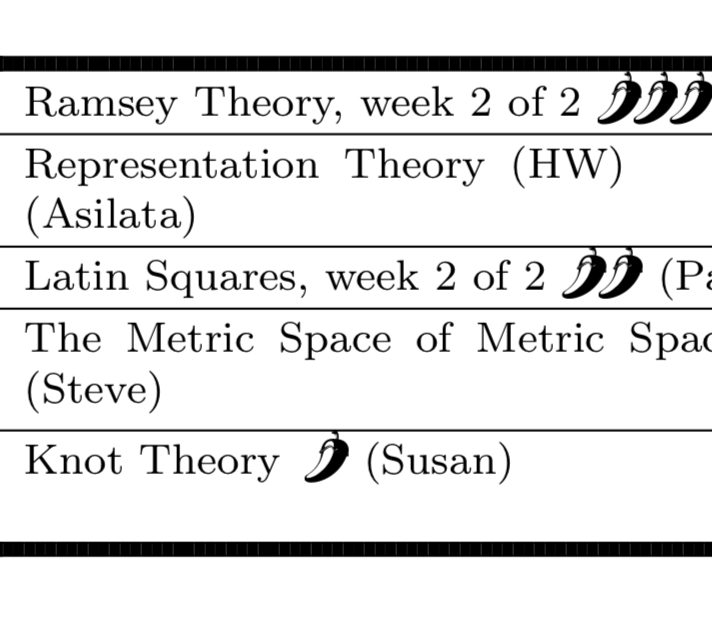## 2012 ClassesHere is an overview of our courses from this summer. You can also see a grid version of the 2012 global schedule.

### Algebra

• Constructible Numbers
• Field Extensions and Galois Theory
• Finite Fields
• Geometry of Surfaces
• Introduction to Group Theory
• Invariant Theory
• Linear Algebra
• Multilinear Algebra
• Representation Theory
• Ring Theory
• Typing with Weird Keyboards
• Unmultiplication

### Analysis

• Asymptotics
• Complex Variables
• Epsilon the Enemy
• Geometric Mapping Theory
• Multivariable Calculus Crash Course
• The Line Without a Length
• The Shape of Infinity
• Things That Will Make You Unhappy
• Vibrations, Waves, Diffusion
• Colloquium: Quaternions in Everyday Life

### Applied Math

• Modelling (An Introduction to Differential Equations)
• Musical Orbifolds
• Quantum Mechanics
• Randomness
• Voting Theory
• Colloquium: Cooperation, Evolution, and Theories of Mind
• Colloquium: Discovery of the Higgs Boson

### Combinatorics

• Caps in the Game SET
• Combinatorial Games
• Crossing Numbers, from 1954 to 2012
• Introduction to Graph Theory
• Knot Theory
• Latin Squares
• Obstacle Numbers of Graphs
• Proof of the 4 Color Theorem
• Quasirandom Graphs
• Ramsey Theory
• Summing Series
• The Alternating Sign Matrix Theorem
• The Alternating Sign Matrix Zoo
• The Dragon Marriage Condition
• The John Conway Hour
• The Probabilistic Method
• What is Grünbaum's Conjecture?
• Colloquium: Parking Functions & Hyperplane Arrangements

### Computer Science

• Five Programming Languages in Ten Days
• Lambda Calculus
• Many Campers Sort Piles
• Colloquium: The Controversy Around the Church–Turing Thesis

### Geometry

• Fractals
• Polytopes
• The Real Projective Plane
• Colloquium: Hearing the Geometry of a Manifold: an Introduction to the Inverse Spectral Problem
• Colloquium: How to Avoid Being Eaten by Bears... in Space!
• Colloquium: Imaginary numbers (aren't they all?)

### Logic/Set Theory

• Computability Theory
• Metric Spaces (of Metric Spaces!)
• Model Theory
• Proof Techniques
• Schemes
• Set Theory: Axioms and Models
• Set Theory: Counting the Uncountable
• The Category of Sets
• The Continuum Hypothesis
• Colloquium: Blocking Light
• Colloquium: Computability of Linear Orderings on Groups

### Number Theory

• Introduction to Number Theory
• Rational Numbers . . . in Space! (Or, Diophantine approximations via geometry)
• Shall We Count?
• Unique Factorization and Fermat's Last Theorem
• Colloquium: Counting Solutions of Polynomial Equations Modulo \$p\$
• Colloquium: Numbers of the form x3 + y3 modulo p

### Problem Solving

• Problem Solving: Inequalities
• Problem Solving: Polynomials
• The AMC Game

### Topology

• The Classification of Surfaces
• The Fundamental Group